# 115 tasks from IT interviews

When a person is invited for a developer position, an employer tests the candidate for both the programming skills and logic tasks, IT cases and tasks on development for professional programmers.

Most commonly, the list of these tasks is the same for many employers, so it means that we can get prepared for any interview.

We decided to create a collection of the most interesting and popular tasks for developers that you can face during the interview.

So, let’s get started!

1. There is a unidirectional list of structures. In it, random points to some other element of the same list. You need to write a function that copies this list with saving the structure (in case the first node’s random pointed to the 4th in the old list, the new list should be the same – the random of the first node points to the 4th node of the new list) . Here you can use O(n), constant additional memory, and memory for the new list elements. It is not allowed to allocate memory for all the data by one block. Thus, the list should be honest, scattered in parts, and not a single block, like an array.

Solution Implementation option

2. A classic task from interviews in Google. You see the numbers on the table, and you should answer the following question: what number goes after?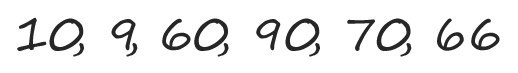Solution

3. Let’s assume you flight from London to Berlin and back, with no wind at all. Afterwards, you make exactly the same flight, but this time there is a constant west wind. During the first direction, you face the tailwind, during the opposite one – frontal.

How will the total flight time of a round trip change?

•  It will decrease

•  It will increase

•  It will not change

Solution

4. What is wrong with this C++ code block?

operator int() const { return *this; }

Solution

5. A task that was popular during the interviews at Amazon earlier. The task is to continue the sequence.

SSS, SSC, SCC, SS

Solution

6. How to calculate it without using a calculator? Can you give an approximate answer?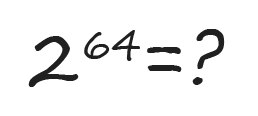Solution

7. “You have been reduced to the size of a 5 cent coin and thrown into a blender. Your weight has decreased so that the density of your body remains the same. Blades will begin to rotate after 60 seconds. What are you going to do?”

This is a classic task asked at Google. You can hardly find a deep analysis of this task around the web, but we have prepared it for you. There is no absolute right answer, but there are those clearly better than others.

Solution analysis

8. Question about C++. What is the error “pure virtual function call”? When it can be output? Provide the minimum code leading to it.

Solution

9. You manage 10 thousand servers in the data center with remote control. You have only one day to get a million dollars. What actions will you perform?

Solution

10. You have an analog clock with a second hand. How many times a day do all three hands overlap each other?Solution

11. What is the difference between string and String in C#?Solution

12. You play football on a deserted island and you want to flip a coin to decide which team will get the ball. The only coin you have is bent, and therefore introduces a clear distortion in the result when tossing. How can you still use such a coin to make a fair decision?

Solution

13. How many golf balls will herd into the school bus?

For reference, the National Transport Vehicle Standards for Schools in the USA for 1995 indicate the maximum size of a school bus – 40 feet long and 8.5 feet wide. The standard diameter of a golf ball is 1.69 inches with a tolerance of 0.005 inches.

Solution

14. Let’s imagine a rotating disc, such as a DVD. You have black (B) and white (W) paints. There is a small sensor that detects the color under it and gives the result as a signal at the edge of the disk. How would you color the disk so that it becomes possible to determine the direction of rotation according to the sensor?

We want to give a brief explanation about the task. The first thing to keep in mind is that you cannot monitor the disk’s rotation. For example, you sit in an office, but the disk rotates in a closed lab. The only way to determine the rotation direction is to use digitized sensor records, and nothing more.

The sensor records the color of the point in the immediate installation site at successive points in time. The testimony is presented in the form of “BBWW…”. The task is reduced to such a coloring of the disk, where the sequence of readings differs when rotating in a straight line and in the opposite direction.

Solution

15. You have the source code of the application written in C, which crashes after launch. After ten starts in the debugger, you find that the program crashes each time in different places of the code. The application is single-threaded and uses only the standard C library. What errors can cause the application crashes? How will you check each one?

Solution

Find errors in the following code block:

unsigned int i; for (i = 100; i >= 0; i) printf(“%d\n”, i);

Solution

16. Can you explain what this code does?

((n & (n – 1)) == 0)

Solution

17. You are given a 100-story building. If the egg is dropped from the Nth floor height (or from a greater height), it will break. If you drop it from any smaller floor, it will not break. You have two eggs. Find the N value using the minimum number of shots.

Solution

18. Let’s continue tasks on C/C++.

What does the keyword volatile mean and when it can be applied? In case you know the formal meaning, try to provide a situation example when volatile will actually be useful.

Solution

19. You have a sorted MxN matrix. Provide an algorithm for finding an arbitrary element in it. Talking about sorted matrix, we mean a matrix with sorted rows and columns.

Solution

20. Create a method that finds the maximum number of two numbers without using if-else or any other comparison operators.

Solution

21. On a deserted highway, the probability of a car appearing in a 30-minute period is 0.95. What is the probability of its occurrence in 10 minutes?

Solution

22. Write the function of summing two integers without using “+” and other arithmetic operators.

Solution

23. You have a fleet of 50 trucks. Each of them is fully fueled and can cover 100 km. How far can you deliver a certain item with their help? What will be the formula if you have N trucks at your disposal?

Actually, geographically it is a place where there are no gas stations. The only place where you can find fuel is the fuel tanks of trucks. You cannot change a truck for a Toyota Prius hybrid passenger car, for example. However, you have an opportunity to leave a truck without fuel and a driver. Finally, the only thing important in this task is to deliver the valuable item as far as possible.

Solution

24. Create the algorithm for finding the million smallest numbers in a set of billion numbers. Let’s assume that computer memory allows you to store the whole billion of numbers. When you come up with any solution, evaluate its effectiveness over time. Maybe you can improve the existing one and find a better solution?

Solution

25. Write a method that will count the number of digits “2” used in the decimal notation of integers from 0 to n (inclusive). The picture is given as a hint to one of the possible solutions.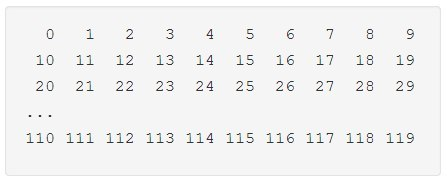Solution

26. Where will you swim faster – in water or syrup?

This is a classic problem with a long history as Isaac Newton had discussed this issue during his lifetime. Recently, it was used during the interviews at Google, but now it is removed. Nevertheless, we recommend you meditate a bit and keep in mind the solution..

Solution

27. Create methods for multiplying, subtracting, and dividing integers, using only the sum operator. The programming language for the implementation is not important. Also, do not worry too much about optimizing the performance and memory usage. The main thing is that you can only use the sum operator. In such tasks, you should recall the essence of mathematical operations.

Solution

28. Suppose you are writing a pipeline where 2 threads process data using the same buffer. The producer thread creates this data, and the consumer thread processes it (Producer – consumer problem). The following code is the simplest model: we create a consumer thread using std::thread, and process data in the main thread.

Let’s omit the synchronization mechanisms of the two threads, and pay attention to the function main(). Try to guess what is wrong with this code and how to fix it?

void produce() { // create the task and put it in the queue } void consume() { // read data from the queue and process it } int main(int , char **) { std::thread thr(consume); // create the thread produce(); // create data for processing thr.join(); // wait for function consume() finish return 0; }

Solution

29. You are given 20 jars of pills. 19 of them are tablets with the 1g weight, and one weighing 1.1 g. You are also given weights that show the exact weight. How to find a jar with heavy pills in one weighing?

Solution

30. An 8×8 chessboard is given. Two opposite catty-corner angles and 31 dominoes were removed. Each domino can close two chessboard squares. Is it possible to fill the whole board with dominoes? Prove your answer.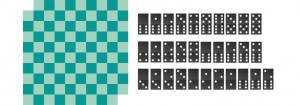Solution

31. You are given the input file containing four billion 32-bit integers. Provide an algorithm that generates a number that is absent in the file. There is 1 GB of memory for this task. Extras: what if you have only 10 MB? The number of passes through the file should be minimal.

Solution

32. Implement an algorithm to print all valid (i.e., properly opened and closed) combinations of n pairs of parentheses.

Solution

33. You put a glass of water on a turntable disc and slowly increase its rotation speed. What will happen earlier:

1) the glass will slide to the side;

2) the glass will tip over;

3) the water will splash.

This question was asked earlier during the interviews at Apple. You need to consider all the possible options and define the main factors for influencing the answer.

Solution

34. A small C++ task for beginners. Why should a polymorphic base class destructor be declared virtual? For your guidance, polymorphic is a class with at least one virtual function.

Solution

35. Write a function that swaps the variables’ values without using temporary variables. Provide as many solutions as you can.

Solution

36. Provide a search algorithm in the single-linked list of the Nth item from the end. The list is implemented manually, there is only one operation to get the next item and a pointer to the first item. The algorithm, if possible, should be optimized in time and memory.

Solution

37. Create a function that defines the number of bits to be changed in order to get an integer from integer A. For example, 32-bit numbers, any language.

This is one of the typical tasks for working with bits quite often asked at interviews. If you have never learned this topic, it will be tough for you to immediately solve the task. For this reason, we recommend you to remember the tools and tricks used in the solution.

Solution

38. The book has N pages, numbered normally from 1 to N. If you sum the numbers of digits included in each page number, the value will be 1095. How many pages are in a book?

Solution

39. Here is a task for validating your C++ knowledge. However, it will be useful for those developers who learn other languages. Match the hash table and the map from the Standard Template Library (STL). How is the hash table organized? What is the optimal data structure for small amounts of data?

Solution

40. Create a class that provides such a locking that prevents the emergence of deadlock.

Solution

41. Create a function in C++ that outputs the last K lines of the file to the standard output stream. The file is very large, say 50 GB, the length of each line does not exceed 256 characters, and the number K  is less than 1000.

Solution

42. You are given a piece of cheese in the shape of a cube and a knife. What is the minimum number of cuts you will need to make to divide this piece into 27 identical cubes? Into 64 cubes? Once cut, you can assemble the parts as you want.

Solution

43. Create a method that determines whether one string is a permutation of another. Talking about permutation, we mean any change in the order of characters. The letter case and spaces are significant.

Solution

44. In the dark room, you are handed a deck of cards. A known number of N cards are face up and the rest are face down. You cannot see the cards, but you can turn them over. How will you divide the card deck into two parts so that each of them has the same number of face up cards?

This task was once popular at interviews in JP Morgan Chase. Surely, once in the dark room, you will just take your smartphone and use the screen or a flashlight. However, this task had created before the era of smartphones and can be solved without observing the cards.

Solution

45. Manually create a stack with standard push/pop functions and an additional min function that returns the minimum stack element. All of these functions should work for O(1). Also, you need to optimize the solution for memory usage.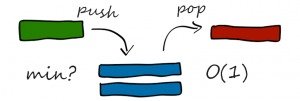Solution

46. How many integers in the range from 1 to 1000 have the number 3? You need to calculate without using a computer.

Solution

47. You have a lot of URLs, about 10 billion. How would you organize an effective duplicate search? Surely, all of them cannot be stored in the memory.

Solution

48. You need to choose one of two bets. In case of the first one, you need to throw the basketball into the basket in one shot. If you succeed, you get 50 thousand dollars. In case of the second option, you need to succeed two times out of three throws, and then you will also receive the same 50 thousand dollars.

Thus, what option would you prefer? Will your ability to throw balls to influence the choice?

Solution

49. Just imagine a triangle made up of numbers. One number is located at the top. Below are two numbers, then three, and so on to the bottom. You start at the top, and you need to go down to the triangle base. Per each move, you can go down for one level and choose between two numbers under the current position. Going through the triangle, you “collect” and summarize the numbers that you pass. Your goal is to find the maximum amount that can be obtained from various passings.

What algorithm can you provide? What will be its complexity and is it possible to provide the best option?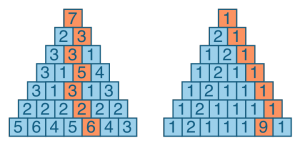Solution

50. Two words or phrases are given, and your task is to check whether they are anagrams.

Anagram is a word game. The main essence is to get another word or phrase by rearranging the letters. Thus, two words are anagrams if we can get one from the other by rearranging the letters.

Solution

51. Provide an algorithm that resets the matrix column N and row M if the element in the cell (N, M) is zero. Surely, you need to provide the most efficient solution improving the memory usage and performance.

Solution

52. Create an algorithm that finds all the array integer pairs if their sum is equal to the set value.

Solution

53. Let’s suppose you need to create an algorithm that demonstrates the person’s contacts’ circle for social networks. How would you build it if you know that the database is very large?

A large base means about a billion registered users and at least 100 billion “friendly” links between them.

Solution

54. Suppose you have a unidirectional list with a loop. Its last element contains a pointer to one of the same list elements, not necessarily the first. Your task is to find the initial loop node.

Elements of the list cannot be changed. You can use only a constant memory.

Solution

55. There is a rule on the island: blue-eyed people cannot be there. The airplane leaves the island every evening at 20:00. All residents gather at the round table every day, each person can see the color of the eyes of other people, but does not know the color of their own. No one has the right to tell a person’s eye color. However, there is at least one blue-eyed person on the island. How many days will it take for all the blue-eyed people to leave?

Solution

56. Write code that removes duplicates from an unsorted linked list. You can only use constant memory.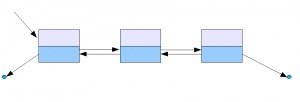Solution

57. Write a method for shuffling a card deck. The deck should be perfectly mixed. It means that card swaps must be equally probable. You can use the perfect random number generator.

Solution

58. Let’s suppose you were assigned the task of developing a search robot. Roughly speaking, it is an application that visits pages on the Internet, indexes, selects links from them, follows them and repeats the process. Here is the question: How to avoid circularity?

Solution

59. You have a glass jug in with the small balls inside. You can define their number at any time. Your friend and you are playing the following game: each of you takes 1 or 2 balls from the jug. The player who takes the last ball wins. What is the best strategy in this game? Can you predict who will win?

Solution

60. There are N companies, and you want them to merge and form one large corporation. How many different ways can you provide them for merging? Absorption stands for a particular cause of merging. For example, A merges B and B is merged by A – two different situations. Equivalent mergers are also possible.

Solution

61. What is the minimum set of coins needed to give any change from 1 to 99 cents? Available coin values ​​are 1, 5, 10, 25, 50 cents and 1 dollar.

Solution

62. You have 25 horses. How many races do you need to determine the three fastest horses? In terms of this task, you cannot use the stopwatch. Only five horses can participate in each race.

Solution

63. Here is a small task for quick wittedness. According to a study, 70% of people love coffee, while 80% love tea. What are the upper and lower proportion bounds of people that love both coffee and tea?

Solution

It is a task that needs to be solved without a calculator and a computer, having only a pencil and paper. How many zeros at the end of factorial 100?

Solution

64. Create an algorithm for finding the largest sum of a continuous sequence from an array of integers, both positive and negative.

Solution

65. Build an application for calculating the value of the median in the numbers’ stream, dynamically tracking the new incoming numbers received by the random number.

Solution

66. It is raining, but you need to get to your car, which stands at the farthest end of the parking lot. Will you run to it or not, if your goal is to get wet as little as possible? How will you behave if you have an umbrella?

Solution

67. Just imagine that there is a square matrix, each pixel of which can be black or white. Develop an algorithm for finding the maximum subsquare where all sides are black.

Solution

68. Let’s suppose that only uncommunicative visitors go to a certain bar. There are 25 chairs along the bar. Whenever a new visitor enters, they necessarily take the furthest seat from the rest of the guests. No one will sit next to someone else: if a visitor enters the bar, but there are no free seats, they immediately turn around and leave the bar. The bartender, naturally, wants to have as many clients as possible along the bar. In case the bartender is allowed to choose the seat for the first visitor, what place will be the most profitable from the point of bartender’s view?

Solution

69. Describe how you can use a single one-dimensional array to implement three stacks.

Solution

70. Suppose you have an unlimited number of coins of 25, 10, 5 and 1 cent. Create a code defining the number of ways to represent n cents.

Solution

71. Write a code that allows you to find the minimum distance (expressed by the number of words) between any two words in the file. The order is completely not important.

Will linear time be enough? How much memory is required to solve the task?

Solution

72. Simulate the use of a dice with seven facets if you only have a dice with five faces.

Put it simply, how to get a random number in the range from 1 to 7, using a generator of random integers from 1 to 5?

Solution

73. Create an algorithm that breaks a coherent list around a value so that all smaller nodes are in front of the nodes greater than or equal to that value.

Solution

74. Create a method that generates a random sequence of M integers from an array of N size. All elements should be selected with the same probability.

The first thing that may come to mind is to select random elements from the initial array and place them in a new array. However, what may happen if we select the same element twice?

Solution

75. Imagine a robot located in the upper left grid corner with coordinates (X, Y). The robot can move in two directions: right and down. How many routes exist from (0, 0) to (X, Y)?

In addition, assume that there are areas on the grid that the robot cannot cross. Create an algorithm for constructing a route from the upper left to the lower right corner.

Solution

76. Create a string compression method based on a duplicate character count. For example, the string aabcccccaaa should turn into a2b1c5a3. If the “compressed” string is longer than the original one, the method should return the original string.

Solution

77. Imagine that you are in a car where a balloon filled with helium is tied to the floor. The windows are closed. You push the gas pedal. What happens to the ball: will it move forward, backward or will it remain in the same position?

What happens to the ball?

• Move back against the movement

•  Move forward in motion

•  Will remain in placeSolution

78. Here is the task through which you can briefly become familiar with the basics of RSA-cryptography.

Let’s suppose you want to make sure that your friend John has your phone number. However, you cannot ask him directly. You will have to write him a message on the card and give the card to Kate, who will act as an intermediary. Kate will carry the card to Petya, he will write a message and give it to Kate, and then she will bring it back to you. However, you do not want Katya to know your phone number. Thus, here is the question: how should you formulate the question to John under existing conditions?

Even without knowing anything about RSA, you can try to come up with a solution.

Solution

79. Here is the task of specific languages’ knowledge. Explain the difference between templates in C++ and generics in Java.

Many programmers believe that C++ templates and generics (for example, in Java) are equal terms, because their syntax is similar: in both cases you can write something like List <T>. However, there are significant differences between these two terms.

Solution

80. Manually create a smart pointer with automatic memory management in C++.

A smart pointer is the same conventional pointer that provides security through automatic memory management. Such a tool helps to avoid many problems: “hanging” pointers, memory leaks and memory allocation failures. The smart pointer should count the number of links to the specified object.

At first sight, this task seems rather complicated, especially if you are not a professional developer in C++.

Solution

81. This task is pretty well-known as the creators try to confuse you by proposing to change your mind. It is also known as the “Monty Hall Paradox”. Monty Hall was the first presenter of the television game show “Let’s make a deal”.

You are given three boxes: one of them contains a valuable prize, others contain nothing. You can choose any box, but you still do not know exactly where is the prize. One of the two boxes you have not selected will be opened and shown that it is empty. Currently, you can either leave the box that you originally chose, or replace it with another, unopened. What do you prefer to do (leave or replace)?

Solution

82. You have an empty room and a group of people outside. Per one iteration, you can either allow one person to enter the room, or release one person from it. Can you provide a series of iterations when every possible combination of people is in the room only once?

It takes time to comprehend what exactly the interviewer wants from you. A simple example will help to understand the task. Say, behind the threshold are two people, Larry and Jim. There are four possible combinations of their presence in the room, considering the case when there is no one in the room at all.

Here these 4 combinations:

• No one in the room.

• Larry is indoors only.

• Jim is indoors only.

• Both Larry and Jim are indoors..

Thus, the question is whether we can start by the first iteration (no one in the room), and then pass through the mentioned above sequence of steps?

Simply put, the question is how to generate non-repeating combinations, changing only one element at a time?

Solution

83. Create the submatrix search code with the maximum possible sum in the N*N matrix containing positive and negative numbers.

Solution

84. Here is a difficult task which requires the ability to create algorithms.

According to the task condition, it is required to create an algorithm that allows to find the Kth number from an ordered number series. There should be only 3, 5 and 7 in the decomposition of elements.

Solution with code examples

85. Given a list of millions of words, design an algorithm to create the largest possible rectangle of letters such that every row forms a word (reading left to right) and every column forms a word (reading top to bottom). The words need not be chosen consecutively from the list but all rows must be the same length and all columns must be the same height.

Solution

86. In this task, you are given an array of integers. You are asked to create a function that receives the source array as input, and returns an array. Each value of the returned array is obtained by multiplying all the values ​​of the original array with a different index.

For your guidance, we provide you with an example. Let’s suppose the source array has the following form:

[1, 7, 3, 4]

Then the function should return:

[84, 12, 28, 21]

The calculation of the values will be the following:

[7 * 3 * 4, 1 * 3 * 4, 1 * 7 * 4, 1 * 7 * 3]

Here are additional conditions for the task:

• It is not allowed to use division.

• The function should be with the least memory and runtime.

Solution

87. The task is specially created for those who love speculating. It is not important to provide an accurate solution, but you need to show the way of thinking. Imagine that you need to get from point A to point B, but you don’t know how to do it. What will you do?

Solution

88. Imagine you are given a task to create a plan of a large city evacuation (let it be San Francisco). Where will you begin?

Solution

89. Here is the task that was asked during the interviews at Apple: you have an array of integers, including negative. You need to find the biggest product of 3 numbers of the array.

Here is an example: you have an array list_of_ints containing the numbers -10, -10, 1, 3, 2. The function that processes this array should return 300, since -10 * -10 * 3 = 300. The task must be performed in the most efficient way. Do not forget to take into account negative numbers.

Solution

90. Let’s imagine a country where all parents want to have a son. Each family continues to give birth to children until they have a boy, and then stops. What will be the ratio of boys and girls in this country?

You should ignore the situations where twins, triplets, couples who have no children, and couples who died before they make a son.

Solution

91. Here is the task for an approximate solution. It is a popular class of tasks that are offered at interviews in an IT company. Here we offer you several tasks, as well as a story about the general methods of solving them and specific advice for interviews.

How many shampoo bottles are produced in the world per year? Answer

How much is 2 to the 64 power? Answer

How much toilet paper will it take to cover the entire state? Answer

How many rubber atoms are erased from a car tire at each turn? Answer

How much money will I need to wash all the windows in Seattle?

Solution

92. Here is the task of merging intervals in the calendar.

Let’s suppose you are working in a company and it is developing an electronic calendar. The calendar has a function that shows when various programming teams will be busy at a meeting.

Those periods when the team is busy are marked on the calendar as time ranges, for example, from 10:00 to 12:30 or from 12:30 to 13:00. In the developed program, the time interval is presented in the form of tuples of two integers. The number indicates the number of the 30-minute block, which comes after 9:00 am. For example, a tuple (2, 4) means a range from 10:00 to 11:00, and (0, 1) is a span of 9: 00-9: 30.

You need to write a function that should simplify the output of information in such a way that if the team is busy from 10:00 to 12:30 and from 12:30 to 13:00, then it was displayed as 10: 00‒13: 00. For example: the input of your function is an unordered array of tuples [(0, 1), (3, 5), (4, 8), (10, 12), (9, 10)], and you must get an ordered array at the output [(0, 1), (3, 8), (9, 12)].

In the future, it is planned to make changes to the program, where instead of the 30-minute blocks there will be minute minutes, as implemented in the Unix-time view. Given this change, you need your function to be able to work with large numbers right now. Do not forget that a tuple is a data type in which the contents of a variable cannot be changed after its creation.

Solution

93. This task was often offered at the interviews at Apple. Imagine you got a job as a cashier in a store. Your boss accidentally found out that you have the development skills, and wanted you to help him create an application.

Input data:

1. Specified amount of money.

2. An array with all available coins’ denominations.

You need to create a function that, at the output, will give the number of all possible ways to get the specified amount of money using the various available coin values. For example, if you need to get 4 cents from coins of nominals of 1, 2 and 3 cents, then the function will return 4 – just as many possible combinations of the numbers 1, 2 and 3 to get in the amount of 4:

1. 1, 1, 1, 1.

2. 1, 1, 2.

3. 1, 3.

4. 2, 2.

Solution

94. This task was once given to Google.

You need to climb the stairs. You can climb one or two steps per iteration. How many ways are there to get to the Nth step?

Solution

95. This task was given at Twitter interviews.

Let’s take a look at the following picture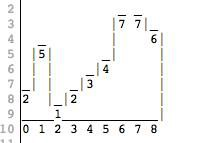This picture shows walls of different heights in a certain flat world. The image is represented by an array of integers, where the index is a point on the X axis. The value of each index is the height of the wall (the value on the Y axis). The picture above corresponds to the array [2, 5, 1, 2, 3, 4, 7, 7, 6].

Now imagine that it started raining, which does not stop and water the walls from above in a steady stream. How much water will gather in the “puddles” between the walls?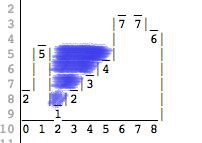The water volume unit is a 1x1x square block. In the picture above, everything that is located to the left of point 1 spills out. Water to the right of point 7 is also spilled. We are left with a puddle between 1 and 6 – so the resulting water volume is 10.

Solution

96. Here is the task about an infinite train.

Imagine a closed railway. A train is traveling along it, the last truck of which is fastened to the first one so that inside you can move freely between the cars. You ended up in one random truck and your task is to calculate their total number. In each truck, you can turn on or off the light, but the initial position of the switches is random and not known in advance.

All the trucks inside look strictly the same, the windows are closed so that it is impossible to look outside, the train moves evenly. It is not allowed to mark trucks in any way other than turning the light on or off.

Solution

97. You are standing in front of a closed room in which there are three light bulbs. There are three switches on the wall: each of them turns on or off a particular light bulb. You need to find out which switch belongs to which light bulb, provided that you can enter the room only once.

The switches are located in a random way. The connection order is not known in advance. Entering the room, you can do anything with light bulbs, except for going back to the switches. At the very beginning, all lamps are turned off.

Solution

98. Here is a classic challenge. You need to find the longest palindrome substring (reads the same backward as forward) in a given string. Provide the most efficient algorithm.

Solution

99. Create a function of square rooting without the programming language integrated means rooting or exponentiation.

Solution

100. In this task, you need to create a function that checks the parity number using only the bit operations AND, OR, NOT.

Solution

101. On the right line, you are are provided with N segments (in real life, these can be periods of time, for example), which are given by the coordinates of their left and right ends. For each given segment, you need to know how much of these segments are completely in it. One segment is fully contained in the second, if the left end of the first segment is to the right of the left end of the second segment, and the right end of the first is to the left of the right end of the second. Offer the most effective way to solve this problem. It is guaranteed that all the ends of these segments are different.

Can you solve this problem effectively if the ends of the segments can coincide?

Solution

102.  Implement an algorithm to determine if a string has all unique characters. What if you cannot use additional data structures?

Solution

103. There is one “magic” index in the array of random numbers A[0…n-1]: such that A[i] = i. The values ​​of the elements in the array can not be repeated. Given that the array is sorted by values ​​in ascending order, create a method that determines this “magic” index, if it exists in array A. If there is no element in the array, return any negative number.

How will the solution change if it is known that there are several such indices in the array?

Solution

104. How can I find out the number of days in a month, knowing its number? In other words, describe how to get the function f(x), which would give the following list of values: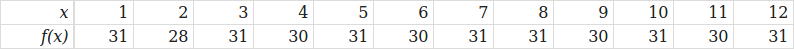As an argument, we get only the number of the month, i.e. we do not consider leap years, and f(2) = 28.

Solution

105. Here is the task for Python developers: you need to go through all the pairs of characters in the string, and stop when you find two identical characters.

The solution is quite obvious, but the question arises:

s = “some string”

for i in range (len (s)):

for j in range (i + 1, len (s)):

if s [i] == s [j]:

print (i, j)

break # How to exit two cycles at once?

For example, If we code in Java, then we can use the tag mechanism:

outterLoop: for (int i = 0; i <n; i ++) {

for (int j = i; j <n; j ++) {

if (/ * something * /) {

break outterLoop;

}

}

}

However, there is no such mechanism in Python. You need to provide the most user-friendly and readable solution.

Solution

106. Here you need to write a code that checks whether two given right line intersect in the same plane.

Let’s suppose that we need to develop a data structure for storing information about a right line, and we assume that if two lines coincide, then they intersect.

Solution

107. Here is the classic task: calculate the Nth number of the sequence in which each element is equal to the sum of the two previous ones. Such a sequence is called a Fibonacci numbers: 1, 1, 2, 3, 5, 8 …

Quite often, at different competitions, the organizators offer similar tasks to confuse you to use a simple sorting. However, if we calculate the number of possible options, we immediately see the ineffectiveness of this approach: for example, the simple recursive function below will consume significant resources on the 30th Fibonacci number, while at competitions, the solution time is often limited to 1-5 seconds.

int fibo (int n)

{

if (n == 1 || n == 2) {

return 1;

} else {

return fibo (n – 1) + fibo (n – 2);

}

}

You need to figure out how to find Nth Fibonacci number in a reasonable time.

Solution

108. Here is one of the most famous tasks around the web, disturbing many bright minds of the world.

The plane stands on the runway with a movable cover like a conveyor. The coating can move against the direction of the airplane, thus towards it. The transporter automatically adjusts the speed so that the plane remains stationary.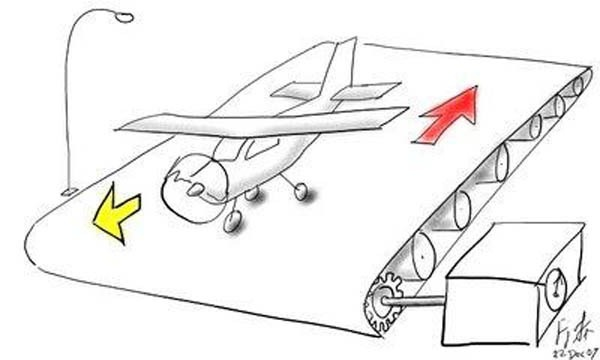The question is whether the plane will take off.

Solution

109. Here is the task of overloading functions in C++, which may be harder than it looks.

Suppose we have two classes:

class Parent {

public:

virtual void print () {

std :: cout << “Parent class” << std :: endl;

}

};

class Derived: public Parent {

public:

virtual void print (int x) {

std :: cout << “Derived class” << std :: endl;

}

};

The question: what will the next two pieces of code display and why?

int main () {

Derived * derived = new Derived;

derived -> print ();

return 0;

}

int main () {

Parent * derived = new Derived;

derived -> print ();

return 0;

}

Solution

110. Let’s consider a situation where three employees want to calculate their average salary, provided that everyone knows their salary. However, they cannot tell it to the other employee directly. The information exchange between these employees is possible, but the messages sent to each other should not contain any specific information about the level of salaries. How to do it?

Solution

111. Imagine you have an array of positive numbers in which all numbers, except three, occur 2 times. These three numbers are different from all the others and each one is found exactly once. You need to find these three numbers. Numbers are placed in a 32-bit integer type.

Solution

112. Let’s suppose we have some finite sequence of numbers and we have an iterator pointing to the first element. We can use the iterator to look at the value of the current element and move on to the next element. It is required to construct such an algorithm for selecting a random element from this sequence so that each element can be selected with equal probability.

The restrictions are the following: we can use O(1) additional memory and cannot create a new iterator. You can use the function of generating a random number from [0; 1).

Solution

113. Here is a well-known task from IT interviews: how to properly implement the exchange of variable values?

Solution

114. There are three people and three lions on one river side. They should all move to another riverside. There is only one boat which can include only two living creatures simultaneously (men or lions). You cannot leave more lions than people on the same river bank, as lions will eat people left in the minority. How will you ferry everyone across the river?

Solution

115. Imagine a situation that you received a stack of one penny coins and the height of the Empire State Building. Can all this money be put in the same room?

At first glance, it may seem this is one of those puzzles to evaluate some absurd number. However, this task is much easier, so think carefully.

Solution

Tags: